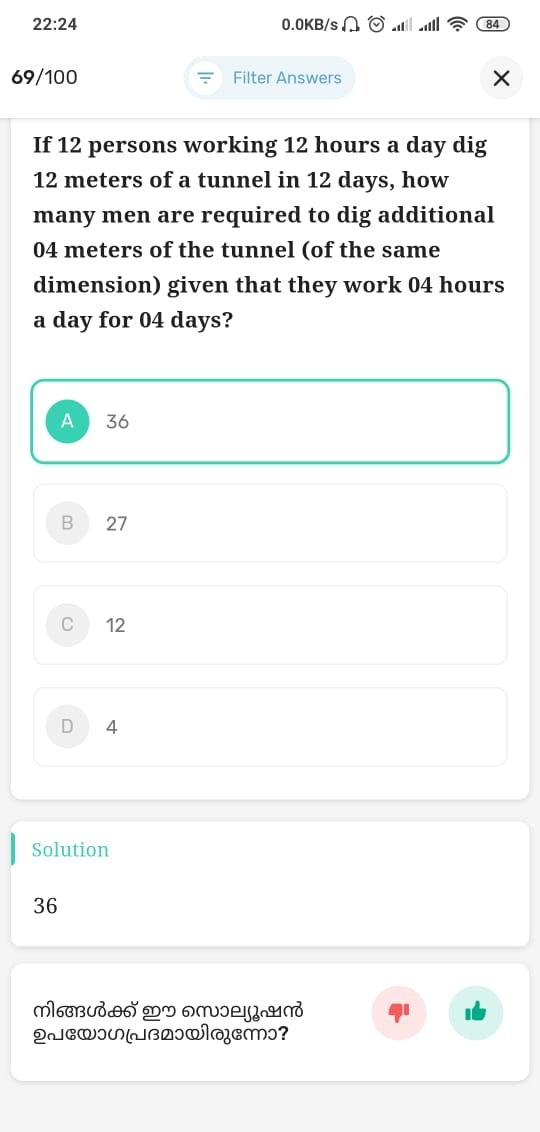R
RAHUL
17 Feb 21

ഇതെങ്ങെനെ solve ചെയ്യുന്നേ, ഇതെന്താ solution illathathReplies to this post

S
suhaira

12 person's12hour for 12 days =12(12)(12). 12 meter tunnel cmplted with (12)(12)(12) hours. so hours to dig1meter tunnel =12(12)(12)/12=(12)(12).1metr needed 144 hours. 4 metre tunnel =4(144). x no of men working 4days 4 hours=4(144). (4)(4)x =4(144). x=144/4=36

0
J
Jayesh

12 person dig 12 m in 12 days 12 person dig 1 m everyday 12 person dig 1/12 m in 1 hour

12 person 1 hour 1 day work = 1/12 m dig 12 person 4 hours of work in 4 days = (1/12)x 4 x4 = 16/12 = 4/3 m

4 m is needed to dig

12 person working 4hrs in 4 days = 4/3 m Find X men working 4 hrs in 4 days = 4 m

4/3 m = 12 4 m = X

X = (12 x 4) x 3/4 = 12x3 = 36

2